Estimation and Comparison of the Weighted Kappa Coefficients of Binary Diagnostic Tests: A Review | OMICS International
Journal of Biometrics & Biostatistics

Like us on:

# Estimation and Comparison of the Weighted Kappa Coefficients of Binary Diagnostic Tests: A Review

José Antonio Roldán Nofuentes*, Juan de Dios Luna Del Castillo and Miguel Angel Montero Alonso

*Corresponding Author:
José Antonio Roldán Nofuentes
Biostatistics, School of Medicine, University of Granada
E-mail: [email protected]

Received date: August 31, 2011; Accepted date: January 13, 2012; Published date: January 18,2012

Citation: Nofuentes JAR, de Dios Luna Del Castillo J, Montero Alonso MA (2012) Estimation and Comparison of the Weighted Kappa Coefficients of Binary Diagnostic Tests: A Review. J Biom Biostat S7:003. doi:10.4172/2155-6180.S7-003

Copyright: © 2012 Nofuentes JAR, et al. This is an open-access article distributed under the terms of the Creative Commons Attribution License, which permits unrestricted use, distribution, and reproduction in any medium, provided the original author and source are credited.

Visit for more related articles at Journal of Biometrics & Biostatistics

#### Abstract

Sensitivity and specificity are classic parameters to assess and to compare the precision of binary diagnostic tests in relation to a gold standard. Another parameter to assess and to compare the performance of binary diagnostic tests is the weighted kappa coefficient, which is a measure of the beyond-chance agreement between the binary diagnostic test and the gold standard, and it is a function of the sensitivity and the specificity of the diagnostic test, the disease prevalence and the relative loss between the false positives and the false negatives. In this study, we carry out a review of the weighted kappa coefficient, its estimation for a single diagnostic test and the hypothesis tests to compare the

weighted kappa coefficients of two or more diagnostic tests, both when the gold standard is applied to all of the subjects in a random sample and when the gold standard is only applied to a subset of subjects in a random sample. The results were applied to different examples.

#### Keywords

Binary diagnostic test; Sensitivity; Specificity; Weighted kappa coefficient

#### Introduction

Diagnostic tests are of fundamental importance in modern medical practice. A diagnostic test is a medical test that is applied to a patient in order to determine the presence of a specific disease. The application of a diagnostic test to assess the presence or absence of a disease has various purposes: a) to provide reliable information about the disease status of a patient; b) to influence to planning of the treatment of a patient; and c) to understand the mechanism and the nature of the disease through research. The interpretation of a diagnostic test depends on several factors: a) the intrinsic ability of the diagnostic test to distinguish between diseased and non-diseased patients (discriminatory accuracy); b) the particular characteristics of each individual; and c) the environment in which the diagnostic tests is applied.

The application of a diagnostic test in the assessment of a disease may lead to errors, and therefore the accuracy of a diagnostic test is measured in terms of probabilities. When the result of a diagnostic test is positive (indicating the provisional presence of the disease) or negative (indicating the provisional absence of the disease), i.e. when the diagnostic test is binary, its accuracy is measured in terms of two probabilities: sensitivity and specificity. Sensitivity (Se) is the probability of a positive result when the individual has the disease, and specificity (Sp) is the probability of a negative result when the individual does not have the disease. The sensitivity and the specificity are the probabilities of obtaining a correct diagnosis of the disease and are the most important parameters to assess the accuracy of a diagnostic test, since they only depend on the biological, physical, chemical, …, bases of the diagnostic test. In order to obtain the unbiased estimators of sensitivity and specificity of the diagnostic test, it is necessary to know the real disease status of each individual in a random sample. The test through which we determine the real disease status is called the gold standard e.g. a biopsy, a clinical assessment, etc. Other classic parameters to assess the performance of a binary diagnostic test are the positive and negative predictive values. The positive predictive value (PPV) is the probability of an individual having the disease when the result of the diagnostic test is positive, and the negative predictive value (NPV) is the probability of an individual not having the disease when the result of the diagnostic test is negative. The predictive values represent the clinical accuracy of the diagnostic test and depend on the sensitivity and the specificity of the diagnostic test and the disease prevalence. Other parameters that are used to assess the accuracy of a binary diagnostic test are the likelihood ratios, which quantify the increase in knowledge of the disease after the application of the diagnostic test and they only depend on the sensitivity and the specificity of the diagnostic test. Therefore, there are several parameters that allow us to assess the performance of a binary diagnostic test in relation to a gold standard.

Another useful parameter to assess the performance of a binary diagnostic test is the weighted kappa coefficient , defined as a measure of the beyond-chance agreement between the diagnostic test and the gold standard. The weighted kappa coefficient of a binary diagnostic test depends on the sensitivity and the specificity of the diagnostic test, on the disease prevalence and on the relative loss between the false positives and the false negatives, and it is a parameter that allows us to assess and compare the performance of binary diagnostic tests in relation to the same gold standard. A review of the use of the weighted kappa coefficient in clinical research can be seen in the work of Kraemer [2,3]. We will now review the main results obtained in the statistical literature related to the weighted kappa coefficient. In Section 2 we study the weighted kappa coefficient of a binary test and its properties. In Section 3 we study the estimation of a binary test through confidence intervals. In Section 4 we study the hypothesis tests to compare the weighted kappa coefficients of two or more binary tests. In Section 5 we study the estimation of the weighted kappa coefficients and the comparison of two or more weighted kappa coefficients in the presence of partial disease verification and in Section we comment on the results.

#### Weighted Kappa Coefficient

Let us consider a binary diagnostic test which is assessed in relation to a gold standard. Let T be the random variable that models the result of the diagnostic test, so that T=1 when the result of the test is positive (indicating the provisional presence of the disease) and T=0 when the result of the test is negative (indicating the provisional absence of the disease); and let D be the random variable that models the result of the gold standard, so that D=1 (positive gold standard) when the individual has the disease and D=0 (negative gold standard) when the individual does not have the disease. Let Se = P (T = 1|D = 1) and Sp = P(T = 0|D = 0) be respectively the sensitivity and the specificity of the diagnostic test, VPP = P (D = 1|T = 1) the positive predictive value and VPN = P(D = 0|T = 0) the negative predictive value, and p = P (D = 1) the disease prevalence. Let L and L' be the losses associated with an erroneous classification with the diagnostic test; L is the loss that occurs when for an individual the diagnostic test is negative and the gold standard is positive, and L’ is the loss that occurs when for an individual the diagnostic test is positive and the gold standard is negative. Losses L and L’ are zero when an individual (diseased or non-diseased) is correctly classified with the diagnostic test. In Table 1 we show the probabilities and the losses associated with the assessment of a binary diagnostic test in relation to a gold standard. In terms of the probabilities and the losses in Table 1, the loss expected when applying the diagnostic test (also known as the “risk of error”  is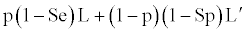Probabilities
T = 1 T = 0 Total
D=1 pSe p(1-Se) p
D=0 (1- p)(1- Sp) (1-p)Sp (1-p)
Total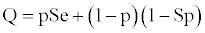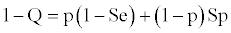1
Losses
T = 1 T = 0 Total
D =1 0 L L
D = 0   0
Total   L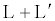Table 1: Probabilities and losses associated with the assessment of a diagnostic test in relation to a gold standard.

and the random loss is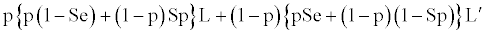The expected loss is the average loss that occurs when erroneously classifying a diseased or non-diseased individual, and its range of values varies between zero and infinite. The random loss is the loss that occurs when the diagnostic test and the gold standard are independent, i.e. when P(T= i|D = j) = P (T= i). In terms of the expected loss and the random loss, the weighted kappa coefficient of a binary diagnostic test is defined as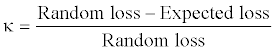and, therefore, is a measure of the relative discrepancy between the random loss and the expected loss, and measures the beyond-chance agreement between the diagnostic test and the gold standard when both are applied to the same set of subjects. The values of the weighted kappa coefficient vary between -1 and 1. Substituting in the previous equation each loss with its corresponding expression it holds that the weighted kappa coefficient of the binary diagnostic test is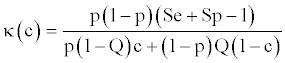where Q = pSe+(1-p)(1-Sp) and c = L (L + L′) is the weighting index. When the loss L is equal to zero, then c = 0 and the weighted kappa coefficient is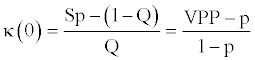and when the loss L' is equal to zero, then c = 1 and the weighted kappa coefficient isThe weighted kappa coefficient can also be written in terms of p,Q, κ (0) and κ (1) as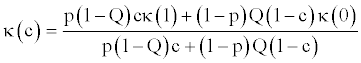and, therefore, the weighted kappa coefficient is a weighted average of κ(0) and κ(1). The weighting index c varies between 0 and 1 and represents the relative loss between the false positives and the false negatives. In practice, the index c is unknown, but its values can be inferred depending on the objective for which the diagnostic test is going to be used. If the diagnostic test is going to be used as a first step towards intensive treatment, there is more concern about false positives and the c index is lower than 0.5; if the diagnostic test is going to be used as a screening test, there is greater concern about false negatives and the c index is greater than 0.5; and c index equals 0.5 when the diagnostic test is used for a simple diagnosis. If L = L' then c = (0.5) and κ (0.5) is called the Cohen kappa coefficient; if L > L' then 0.5 < c < 1, and if L' > L then 0 < c < 0.5. The weighted kappa coefficient of a binary test has the following properties:

• If the classificatory agreement between the binary test and the gold standard is perfect (Se = Sp = 1) then the expected loss is 0 and κ (c) = 1.

• If the sensitivity and the specificity are complementary (Se = 1- Sp), which indicates that the test is independent of the “gold standard”, then κc = 0.

• If the random loss is greater than the expected loss then κc> 0; and if the random loss is lower than the expected loss then κc < 0 and the results of the diagnostic test must be interchanged T=1 must be the negative result and T = 0 must be the positive result). Therefore, the analysis must be limited to the positive values of the weighted kappa coefficient, and its values can be classified in the following scale : from 0 to 0.20 the classificatory agreement is slight, from 0.21 to 0.40 the classificatory agreement is fair, from 0.41 to 0.60 the classificatory agreement is moderate, from 0.61 to 0.80 the classificatory agreement is substantial and from 0.81 to 1 the classificatory agreement is almost perfect.

• The weighted kappa coefficient is a function of the c index which is increasing if Q > p, decreasing if Q < p or constant and equal to Se + Sp - 1 if Q = p.

Once we have defined and analyzed the properties of the weighted kappa coefficient of a binary test, this is a valid parameter to assess and compare the performance of binary diagnostic tests when considering the losses associated with the classification of subjects with binary diagnostic tests in relation to the same gold standard. Then we study the estimation and the comparison of weighted kappa coefficients.

#### Estimation of the Weighted Kappa Coefficient

When the binary diagnostic test and the gold standard are applied to all of the subjects in a random sample sized n we obtain Table 2. In this situation, the maximum likelihood estimators (MLE) of the sensitivity and the specificity of the diagnostic test and the disease prevalence are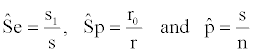T = 1 T = 0 Total
D =1 s1 s0 s
D = 0 r1 r0 r
Total n1 n0 n

Table 2: Frequencies observed when applying the binary test and the gold standard to a random sample sized n.

and the MLE of the weighted kappa coefficient is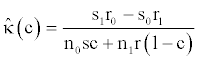with 0 < c <1. As the weighted kappa coefficient is a function of the accuracy of the diagnostic test and the disease prevalence, applying the delta method the estimated variance of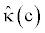is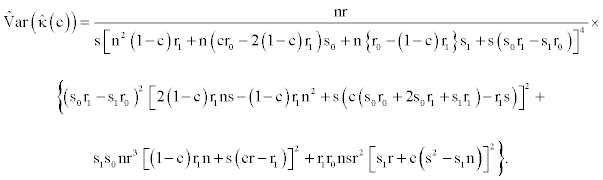Confidence intervals

Roldán Nofuentes et al.  have studied different confidence intervals for the weighted kappa coefficient. Depending on the sample size we can use the following confidence intervals:

1) Wald confidence interval: Assuming the asymptotic normality of, the 100(1- α)% confidence interval for the weighted kappa coefficient is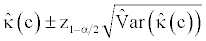where z1-α/2 is the percentile of the normal standard distribution. This confidence interval performs well for relatively small samples (n = 100).

2) Logit confidence interval: Assuming the asymptotic normality ofthe logit transformation of,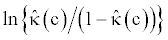follows a normal average distribution ln ln{k(c)/(1-k(c)}. Thus, the 100(1-α)% confidenceinterval for the logit of k(c) is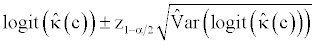wherethe estimator of the variance of the logit of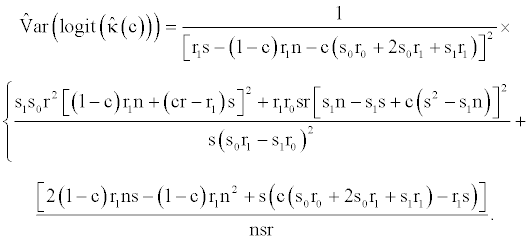Finally, the logit confidence interval for the weighted kappa coefficient is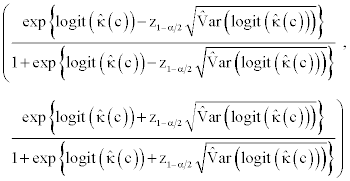This confidence interval performs well for samples of 200 or more.

Example

The results obtained were applied to the study of Weiner et al.  concerning the diagnosis of coronary artery disease, using as a diagnostic test a stress exercise test and as a gold standard a coronary arteriography. In Table 3 we show the results obtained by Weiner et al. for subjects with angina and the estimation of the weighted kappa coefficient for different values of the c weighting index, and where the variable T models the result of the exercise test and the variable D the result of the coronary angiography. From these results it holds that if the exercise stress test is used before an intensive treatment (0 < c < 0.5), the weighted kappa coefficient has an intermediate value for each value of c and the classificatory agreement between the diagnostic test and the gold standard is mainly moderate; if the exercise stress test is used for a simple diagnosis (c = 0.5), the beyond-chance agreement between the test and the gold standard varies between fair and moderate; and if the exercise stress test is used as a screening test (0.5 < c < 1), the beyondchance agreement between the exercise stress test and the coronary angiography is mainly fair.

Frequencies observed
T=1 T =0 Total
D=1 473 81 554
D=0 22 44 66
Total 495 125 620
Estimations of the weighted kappa coefficient
c95% logit CI
0.1 0.527 (0.408, 0.639)
0.2 0.462 (0.370, 0.584)
0.3 0.412 (0.338, 0.539)
0.4 0.371 (0.310, 0.502)
0.5 0.338 (0.285, 0.471)
0.6 0.310 (0.264, 0.444)
0.7 0.287 (0.245, 0.420)
0.8 0.267 (0.229, 0.399)
0.9 0.249 (0.214, 0.380)

Table 3: Data from the study of Weiner et al and estimations of the weighted kappa coefficient.

#### Comparison of Weighted Kappa Coefficients

The comparison of the accuracy of binary diagnostic tests is a topic of special importance in the study of statistical methods for diagnosis. When comparing the accuracy, measured in terms of sensitivity and specificity, of two binary diagnostic tests, this consists of the comparison of binomial proportions through exact or asymptotic methods. In the case of the weighted kappa coefficient, Bloch  studied the comparison of the weighted kappa coefficients of two binary tests in paired designs, and Roldán Nofuentes and Luna del Castillo  generalized the method of Bloch to the case of more than two binary tests.

Comparison of two weighted kappa coefficients

Bloch  studied the comparison of the weighted kappa coefficients of two binary diagnostic tests when the two tests and the gold standard are applied to all of the subjects in a random sample sized n. In this situation we obtain Table 4, where the variable T1 models the result of Test 1, T2 models the result of Test 2 and D the result of the gold standard, and in (Table 5) we show the probabilities associated with each cell of the table of frequencies.

 T1= 1 T1 = 0 T2=1 T2=0 T2=1 T2=0 Total D=1 s11 s10 s01 s00 s D=0 r11 r10 r01 r00 r Total N11 n00 n01 n00 n

Table 4: Frequencies observed when comparing two binary tests in paired designs.

 T1=1 T1=0 T2=1 T2=0 T2=1 T2=0 D = 1 p11 p10 p01 p00 p D = 0 q11 q10 q01 q00 q p11+q11 p10+q10 p01+q01 p00+q00 1

Table 5: Probabilities associated with the comparison of two binary diagnostic tests in paired designs.

Let (η = s11,s10,s01,s00,r11,r10,r01,r00)T and π = (p11,p10,p01,p00,q11,q10,q01,q00)T be two size 8 vectors. In terms of the components of vector π, the weighted kappa coefficient of Test 1 is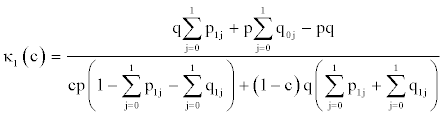and that of Test 2 is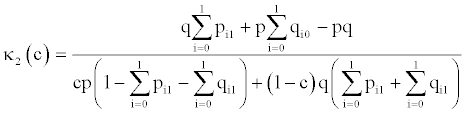As π is a vector of probabilities of a multinomial distribution, its MLE is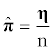and the variance-covariance matrix of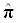is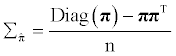Substituting in the expressions of the weighted kappa coefficients each parameter with its MLE, the MLEs of κ1 (c) and κ2 (c) are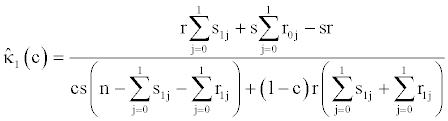and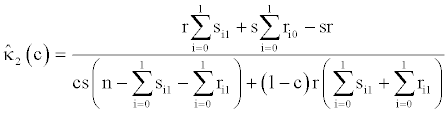respectively. Let vector κ = (κ1(c), κ2=(κ1(c))T, applying the delta method the asymptotic variance-covariance matrix of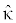is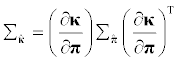Carrying out the algebraic operations and substituting each parameter with its MLE, the estimated asymptotic variances and covariances of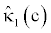and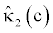are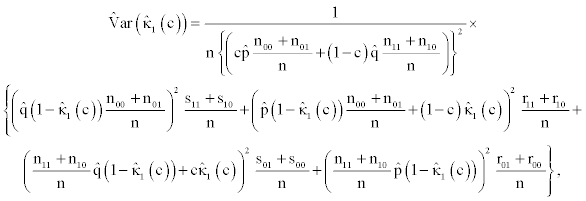and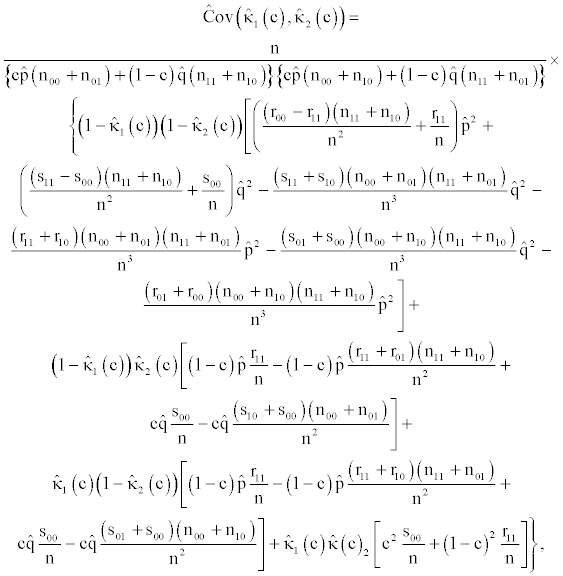where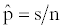and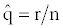For the same value of the weighting index c, the statistic to check the equality of the two weighted kappa coefficients,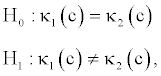is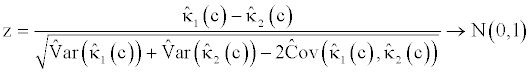This hypothesis test performs well in terms of the type I error and power. In general terms, its type I error fluctuates around the nominal error (even in relatively small samples, e.g. n =100), and with samples of n ≥ 500 the power is high (higher than 80%).

Extension to multiple diagnostic tests

Roldán Nofuentes and Luna del Castillo  generalized the method of Bloch  to the case of more than two diagnostic tests, studying a joint hypothesis test to simultaneously compare the weighted kappa coefficients of more than two binary diagnostic tests in relation to the same gold standard. If we consider J diagnostic tests (J ≥ 3) and a gold standard is applied to all of the subjects in a random sample sized n, and the random Tj models the result of the j-th diagnostic test, the maximum likelihood estimator of the weighted kappa coefficient of the j-th binary test is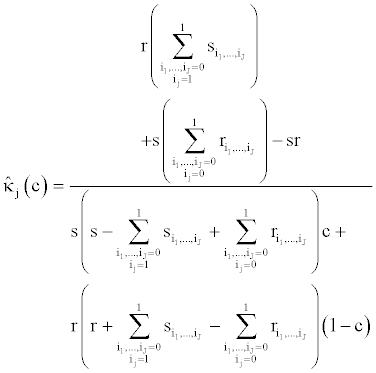when Si1 ,...,iJ s is the number of diseased subjects for whom T1=i1, T2=i2,..., TJ=iJ, with ij=0,1 yj=1,…,J; i1 ,...,iJ r is the number of non-diseased subjects for whom T1=i1, T2=i2,..., TJ = iJ;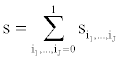is the total number of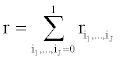diseased subjects; and n = s + r.

Let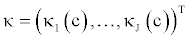be a vector whose components are the J weighted kappa coefficients and let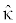the MLE of κ. Let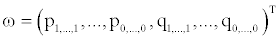be the dimension vector 2J+1 whose components are the probabilities of each cell of the multinomial distribution, and the variance-covariance matrix of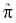is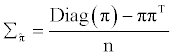As the vector κ(c) is a function of the probabilities of the vector ω, applying the delta method the asymptotic variance-covariance matrix of the vectoris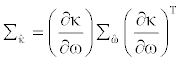and applying the central theorem of the multivariate limit it is verified that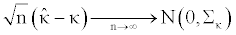For the same value of the weighting index c, the global hypothesis test to contrast the equality of the J weighted kappa coefficients is

H0: κ1(c) = κ2(c) = … = κJ (c)

H1: a least one equality is not true.

This hypothesis test is equivalent to the hypothesis test

H0: φκ = 0

H1: φκ≠ 0,

where φ is a complete range matrix (J -1)×J whose elements are known constants. Thus, for three diagnostic tests? (J =3),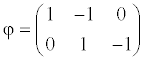Asis distributed asymptotically according to a normal multivariate distribution, the statistic for the global hypothesis test of equality of the J weighted kappa coefficients is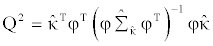and it is distributed asymptotically according to a central chi-square distribution with J -1 degrees of freedom. Roldán Nofuentes and Luna del Castillo  found that for three binary diagnostic tests, in general the joint hypothesis test performs better in terms of type I error and power for samples of at least 500 subjects (the type I error fluctuates around the nominal error and the power is higher than 80%). When the global hypothesis test is significant to an error rate α, investigation into the causes of the significance is done solving the paired comparisons of diagnostic tests applying the method of Bloch  along with Bonferroni correction (carrying out each hypothesis test to an error rate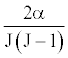or another similar method of multiple comparison. For J =2 the joint hypothesis test is equivalent to the method of Bloch .

Example

Weiner et al.  studied the diagnosis of coronary disease in a sample of 1465 men using as diagnostic tests a exercise stress test and their clinical history and as the gold standard a coronary angiography. In Table 6 we show the results obtained by Weiner et al. and the results obtained when comparing the weighted kappa coefficients of the two diagnostic tests for different values of the c index, and where the variable T1 models the result of the exercise stress test, T2 models the result of the clinical history of the individual and D is the result of the coronary angiography.

Frequencies observed
T1=1 T1=0
T2=1 T2=0 T2=1 T2=0 Total
D = 1 786 29 183 25 1023
D = 0 69 46 176 151 442
Total 855 75 359 176 1465
Comparison of the two weighted kappa coefficients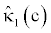H0:k1(c)= k2(c)
c     z p-value
0.1 0.57 0.35 6.35 <10-8
0.2 0.55 0.37 5.38 <10-6
0.3 0.54 0.39 4.26 <10-8
0.4 0.52 0.42 3.04 0.0023
0.5 0.51 0.45 1.77 0.0767
0.6 0.49 0.48 0.31 0.7566
0.7 0.48 0.52 1.24 0.2150
0.8 0.47 0.57 2.92 0.0035
0.9 0.45 0.62 4.71 <10-4

Table 6: Data from the study by Weiner et al and results obtained comparing the weighted kappa coefficients.

From the results obtained when applying the Bloch method  it holds that if both diagnostic tests applied before intensive treatment (0 < c < 0.5) the weighted kappa coefficient of the exercise stress test is significantly higher than that of the clinical history, and therefore the beyond-chance agreement between the exercise stress test and the gold standard (the beyond-chance agreement is moderate) is significantly higher than the beyond-chance agreement between the clinical history and the gold standard (the agreement is fair). If the two diagnostic tests are applied as screening tests (0.5 < c < 1), for c equal to 0.6 and 0.7 no significant differences were found between both weighted kappa coefficients, and for c equal to 0.8 and 0.9 the weighted kappa coefficient of the clinical history is significantly larger than that of the exercise stress test, and therefore the beyond-chance agreement between the clinical history and the gold standard (the beyond-chance agreement varies between moderate and substantial) is significantly larger than the classificatory agreement between the exercise stress test and the gold standard (the agreement is moderate). For a simple diagnosis (c = 0.5) no significant differences were found between the two Cohen kappa coefficients (although there is evidence of significance), and in both cases the beyond-chance agreement is moderate.

#### Weighted Kappa Coefficient in the Presence of Partial Verification

In Sections 3 and 4 we have considered the gold standard being applied to all of the subjects in a random sample sized n. Nevertheless, in clinical practice it is frequent not to apply the gold standard to all of the individuals in the sample, leading to the problem known as partial disease verification . Thus, if the gold standard consists of an expensive test or involves some risk for the individual, the gold standard is not applied to all of the subjects in the sample. This situation corresponds to two-phase studies: in the first phase, the diagnostic test is applied to all of the subjects in the sample, and in the second phase the gold standard is only applied to a subset of subjects in the sample. If in the presence of partial disease verification the sensitivity and the specificity of the diagnostic test are estimated without considering the subjects to whom the gold standard has not been applied, the estimators obtained are affected by so-called verification bias [9-11]. In an analogous way, the weighted kappa coefficient of the diagnostic test cannot be estimated only considering those subjects to whom the gold standard has been applied since the estimator obtained is also affected by verification bias . This same situation of partial disease verification may also appear when comparing accuracy and, therefore, the weighted kappa coefficients, of two or more binary diagnostic tests. We then studied the estimation of the weighted kappa coefficient of a binary test and the comparison of the weighted kappa coefficients of two binary tests in the presence of partial disease verification.

Estimation of the weighted kappa coefficient

When a diagnostic test is applied to a random sample of n subjects and the gold standard is only applied to a subset of these n subjects we obtain Table 7, where T and D are the variables defined in Section 2 and V is the random binary variable that models the verification process, so that V =1 when the disease status of the individual is verified with the gold standard and V =0 when the disease status is not verified with the gold standard it is not known whether or not the individual is diseased. In this table one can observe that there are uj subjects for whom T = j and they are not verified with the gold standard (V= 0) and, therefore, it is not known if they are diseased or not, with j= 0,1. In this situation the verification probabilities λij are defined as the probability of selecting an individual to verify his or her disease status with the gold standard when D= i and T = j, i.e. λij = P (V = 1|D = i,T = j), with i, j = 0,1. If λij = λj for i, j = 0,1, then the verification process does not depend on the disease status and the mechanism of missing data is ignorable . The fact that the mechanism of missing data is ignorable means that the missing data are missing at random , and the parameters of the diagnostic test can be estimated through the method of maximum likelihood.

 T = 1 T = 0 V = 1 D = 1 s1 s0 D = 0 r1 r0 V = 0 u1 u0 Total n1 n0

Table 7: Frequencies observed in the presence of partial disease verification.

When the mechanism of missing data is ignorable, the MLEs of the sensitivity and the specificity of the diagnostic test [9,10] are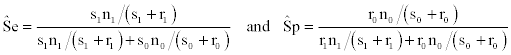and that of the prevalence is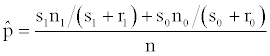where n = n1 + n0, so that the MLE of the weighted kappa coefficient of the diagnostic test  is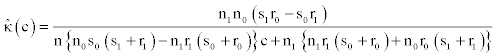Applying the delta method the estimator of the variance ofis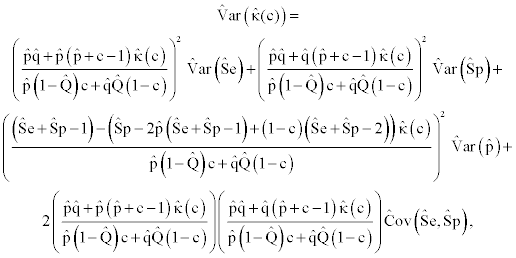where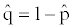, and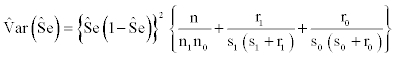,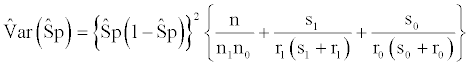,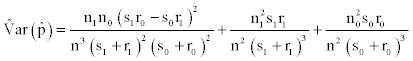,

and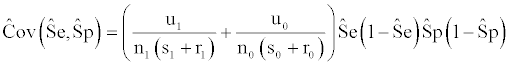Finally, the confidence interval to 100(1 - α)% for theweighted kappa coefficient in the presence of partial disease verification isIn general terms, depending on the verification probabilities, this confidence interval performs well in terms of coverage when the sample size is large (n ≥ 500).

Example

The results of the previous Section were applied to the study of Drum and Christacopoulos  on the diagnosis of hepatic diseases using as the diagnostic test a gammagraphy and as the gold standard a biopsy. In (Table 8) we show the results obtained by Drum and Christacopoulos, the estimated weighted kappa coefficients and the 95% confidence intervals for different values of the weighting index c assuming that the mechanism of missing data is ignorable, and where the variable T models the result of the gammagraphy and the variable D the result of the biopsy. From these results, it holds that if the gammagraphy is used as a screening test as a first step towards intensive treatment, the beyond-chance agreement between the gammagraphy and the biopsy varies between moderate and substantial.

 Frequencies observed T =1 T = 0 Total V= 1 D = 1 231 27 258 D = 0 32 54 86 V= 0 166 140 306 Total 429 221 650 Estimations of the weighted kappa coefficient c95% CI 0.1 0.594 (0.489 , 0.699) 0.2 0.584 (0.482 , 0.686) 0.3 0.575 (0.475 , 0.676) 0.4 0.567 (0.467 , 0.667) 0.5 0.558 (0.457, 0.659) 0.6 0.550 (0.447 , 0.652) 0.7 0.542 (1.437, 0.647) 0.8 0.534 (0.426 , 0642) 0.9 0.526 (0.412 , 0.637)

Table 8: Data from the study of Drum and Christacopoulos and estimations of the weighted kappa coefficient.

Comparison of two weighted kappa coefficients

Roldán Nofuentes and Luna del Castillo  studied the comparison of the weighted kappa coefficients of two binary diagnostic tests in the presence of partial disease verification. If two binary diagnostic tests are applied to all of the subjects in a random sample sized n and the gold standard is only applied to a subset of subjects in the sample Table 9 is obtained, where the variables T1, T2, and D are the variables defined in Section 4.1 and V is the variable defined in Section 5.1. Zhou  studied the comparison of the sensitivities (specificities) of two binary diagnostic tests in the presence of partial disease verification when the mechanism of missing data is ignorable, demonstrating that the comparison of these parameters cannot be carried out neglecting those subjects who are not verified with the gold standard. For the same reason, in the presence of partial verification, the comparison of the weighted kappa coefficients cannot be carried out applying the method of Bloch , since the estimators obtained would be affected by verification bias .

 T1 =1 T1 = 0 T2 =1 T2=0 T2 =1 T2 =0 Total V= 1 D=1 s11 s10 s01 s00 s D=0 r11 r10 r01 r00 r V=0 u11 u10 u01 u00 u Total n11 n10 n01 n00 n

Table 9: Frequencies observed when comparing two binary tests in the presence of partial verification.

When the mechanism of missing data is ignorable, it is verified that the probability of verifying an individual with the gold standard only depends on the results of the two diagnostic tests and not on the disease status i.e.,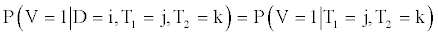with i, j, k = 0,1, then the MLEs of the weighted kappa coefficients of binary tests 1 and 2 are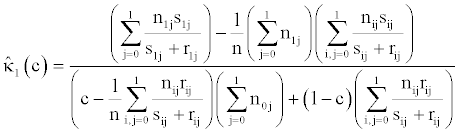and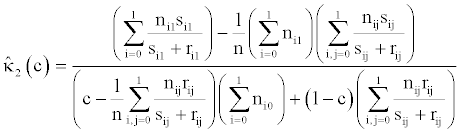respectively. The estimation of the asymptotic variance-covariance matrix of and is obtained applying the delta method (applying a similar procedure to that carried out in Section 4.1). Finally, for the same value of the weighting index c, the statistic to check the equality of the two weighted kappa coefficients,

H0: κ1(c) = κ2(c) H1: κ1(c) ≠ κ2(c),

is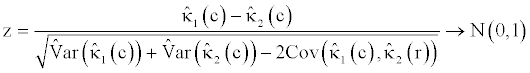Simulation experiments showed that, in general terms, the type I error of this hypothesis test fluctuates around the nominal error for samples with n ≥ 500 (for smaller samples the hypothesis test is conservative) and large samples are needed, between 500 and 1000 subjects depending on the verification probabilities and on the disease prevalence, so that the power is high (higher than 80%).

The method proposed by Roldán Nofuentes and Luna del Castillo  to compare the weighted kappa coefficients of two binary tests in the presence of partial disease verification can be generalized to more than two binary tests , following a similar procedure to that used in Section 4.2.

Example

The results of the previous Section were applied to the study by Hall et al.  on the diagnosis of Alzheimer’s disease, using as diagnostic tests a new test and the classic test and as the gold standard a clinical assessment. In Table 10 we show the data from the study by Hall et al. for people aged 75 and over and the results obtained when comparing the weighted kappa coefficients of the two diagnostic tests for different values of the weighting index c (assuming that the verification process is ignorable), and where the variable T1 models the result of the new diagnostic test, T2 models the result of the classic test and D the result of the gold standard.

Frequencies observed
T1=1 T1=0
T2=1 T2=0 T2=1 T2=0 Total
V= 1
D=1 31 5 3 1 40
D=0 25 10 19 55 109
V=0 22 6 65 346 439
Total 78 21 87 402 588
Comparison of the two weighted kappa coefficients
H0:k1 (c) =k2 ( c )
cz p-value
0.1 0.46 0.26 3.12 0.0018
0.2 0.47 0.28 2.91 0.0036
0.3 0.49 0.30 2.67 0.0076
0.4 0.51 0.33 2.38 0.0173
0.5 0.53 0.37 2.06 0.0394
0.6 0.55 0.40 1.70 0.0891
0.7 0.58 0.45 1.31 0.1902
0.8 0.61 0.52 0.86 0.3898
0.9 0.64 0.60 0.32 0.7490

Table 10: Data from the study by Hall et al and results obtained when comparing the two weighted kappa coefficients.

From the results obtained when applying the method of Roldán Nofuentes and Luna del Castillo  it holds that if both diagnostic tests are applied before intensive treatment (0 < c < 0.5) the weighted kappa coefficient of the new test is significantly larger than that of the classic test, and therefore the beyond-chance agreement between the new test and the gold standard (the beyond-chance agreement is moderate) is significantly larger than the beyond-chance agreement between the classic test and the gold standard (the beyond-chance agreement is fair). If the two diagnostic tests are applied as screening tests (0.5 < c < 1), no significant differences are found between both weighted kappa coefficients (although for c equal to 0.6 there are signs of significance) and, therefore, no significant differences are found between the beyond-chance agreements of each diagnostic test with the gold standard (the beyond-chance agreement varies between moderate and substantial). For a simple diagnosis (c = 0.5) the conclusions are the same as for 0 < c < 0.5.

#### Conclusions

The weighted kappa coefficient is a measure of the classificatory agreement beyond-chance agreement between the diagnostic test and the gold standard, and is a very useful measurement to assess and compare binary diagnostic tests in relation to a gold standard. Although the sensitivity and the specificity, the likelihood ratios and the predictive values are the most common measures to assess and compare the performance of binary diagnostic tests, the weighted kappa coefficient is the measure that should be used in order to assess a binary diagnostic test (or in order to compare two or more binary tests) when considering the losses associated with an erroneous classification using the diagnostic test, and it provides valuable information to understand the classification mechanism of the binary diagnostic test. In this manuscript we have studied in a summarized form the main contributions made by the literature in relation to this parameter, estimating through confidence intervals for a single binary test and checking hypotheses to compare several weighted kappa coefficients, paying special attention to its applications to real medical examples, from two sample situations: when all of the subjects in a random sample are verified with the gold standard and when only a subset of the subjects in the sample are verified with the gold standard (partial disease verification). In the latter situation, when the verification process depends on variables that are related to the disease, the mechanism of missing data is not ignorable and the methods proposed cannot be applied.

When the diseases status of all of the patients is known, the estimation of the weighted kappa coefficient (and the comparison of two or more weighted kappa coefficients) is carried out based out on a transversal design (applying the diagnostic test and the gold standard to all of the subjects in a random sample), just as has been done with the examples from Sections 3.2 and 4.3. If the sampling is retrospective, it is not possible to estimate the disease prevalence and, therefore, it is not possible to estimate the weighted kappa coefficient. Therefore, if the sampling is retrospective, it is not possible either to estimate or compare weighted kappa coefficients (unless the disease prevalence is known or one of its estimators is obtained from another study).

Finally, all of the methods proposed are asymptotic and are based on the asymptotic normality of the estimators of the weighted kappa coefficients, so that, in general, when the sample sizes are small (n < 100) they do not normally perform well.

#### Acknowledgements

TThis research was supported by the Spanish Ministry of Science, Grant Number MTM2009-08886, and the Department for Innovation, Science and Business of the Autonomous Government of Andalusia, Spain, Grant Number FQM-01459. The authors would like to thank Dr. Meijuan Li for her invitation to write this article for the special edition of “Medical statistics: Clinical and experimental research” in the “Journal of Biometrics & Biostatistics”. We thank the referees, editor associate (Dr. Herber Pang) and editor of the “Journal of Biometrics & Biostatistics” for the revision of this manuscript.

#### References

Select your language of interest to view the total content in your interested language

### Article Usage

• Total views: 16690
• [From(publication date):
specialissue-2012 - Dec 06, 2019]
• Breakdown by view type
• HTML page views : 12852Can't read the image? click here to refresh